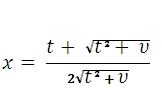# SQL Server T.INV function

Updated: 9 August 2010

Use TINV to calculate the t-value of the Student's t-distribution as a function of the probability and the degrees of freedom. The cumulative distribution function which is given by the incomplete beta function:WhereSyntax
SELECT [wctStatistics].[wct].[TINV] (
<@Probability, float,>
,<@Degrees_freedom, float,>)
Arguments
@Probability
is the probability associated with the two-tailed Student's t-distribution. @Probability is an expression of type float or of a type that can be implicitly converted to float
@Degrees_freedom
is a number specifying the degrees of freedom. @Degrees_freedom is an expression of type float or of a type that can be implicitly converted to float
Return Types
float
Remarks
·         If @Degrees_freedom  < 1, TINV returns an error.
·         @Degrees_freedom is truncated to zero decimal places.
·         If @Probability < 0 or @Probability > 1, TINV returns an error
·         TINV uses root-finding algorithms and iteration to solve for TDIST(TINV(@Probability, @Degrees_freedom),@Degrees_freedom, 2) - @Probability = 0to eight decimal places
Examples

Select
wct.tinv(0.0546449297129976, 60)

This produces the following result

----------------------
1.95999999799997

(1 row(s) affected)

Select
Round(wct.TDIST(wct.tinv(0.0546449297129976, 60), 60, 2) - 0.0546449297129976, 9)

This produces the following result

----------------------
0

(1 row(s) affected)

### SupportCopyright 2008-2023 Westclintech LLC         Privacy Policy        Terms of Service